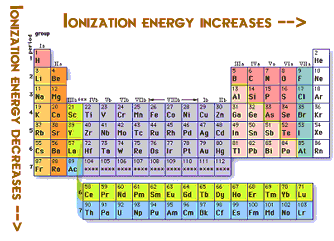# Question #287c9

May 4, 2017

4 for a semi conductor, less than that for a conductor, more for a insulator.

May 4, 2017

Because the ionisation/ionization energy is low for metals than non metals

#### Explanation:

The ionisation/ionization energy is the energy required to remove one valence electron from an atom.

$X + \text{energy} = {X}^{+} + {e}^{-}$

This amount of energy is known as the ionization energy.
If IR is low then the electron can be freed by less energy and if the ionization energy is high the electron can be only freed by high energy.

This also affects the work function of the material and thus work function of metals are lower than that of nonmetals.

In the photoelectric effect this energy is in the form of photons.
When the photon of sufficient energy hits the surface an electron is ejected.The photon must have the energy which is the ionisation energy.So you can see that the ionization energy increases and the very good reason isYou can see that the atomic radius decreases as you move towards the non metals. Thus the force of attraction from the nucleus is more. Moreover the weight of protons and neutrons are more than electrons but this is a very negligible force but still accepted by many physicists.

So a electron in a metal can be easily moved thus creating electric current in the presence of electric field.While in a nonmetal it can't because the IR is too high. And as more valence electrons make the element less reactive it would be hard to remove an electron from a a element with 8 valence electrons and very easy to remove an electron from 5 valence electrons

When many atoms combine to make a solid the orbitals combine and overlap to form a solid. The Pauli exclusion principle states that in a molecule no two electrons can stay in one energy level. When these form the energy levels are so close to each other that they are called energy bands
Moreover the band gaps in the metals are of very less distance and thus electrons can jump to the the conduction band but in insulator the distance is too much while in semiconductors it is intermediate of metals and semiconductors.Though semiconductors need some external energy (thermal) to jump to the conduction band.